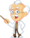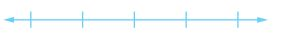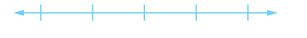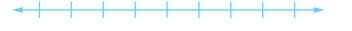Homework Explained - Math Practice 101Dear guest, you are not a registered member. As a guest, you only have read-only access to our books, tests and other practice materials.

As a registered member you can:

 Write an integer for each situation. Explain the meaning of zero in each situation. 15-yard gain    Explain:(show solution) loss of 2 hours    Explain:(show solution) Graph each integer or set of integers on a number line. Question 3 -2(show solution) Question 4 (-1,1,0)(show solution) Question 5 The data set (+5,0,-15,+20) shows the number of points Delaney scored on each hand of a card game.Graph the scores.Explain the meaning of zero in this situation.(show solution) Question 6 Building on the Essential Question How can you use integers to represent data?(show solution)